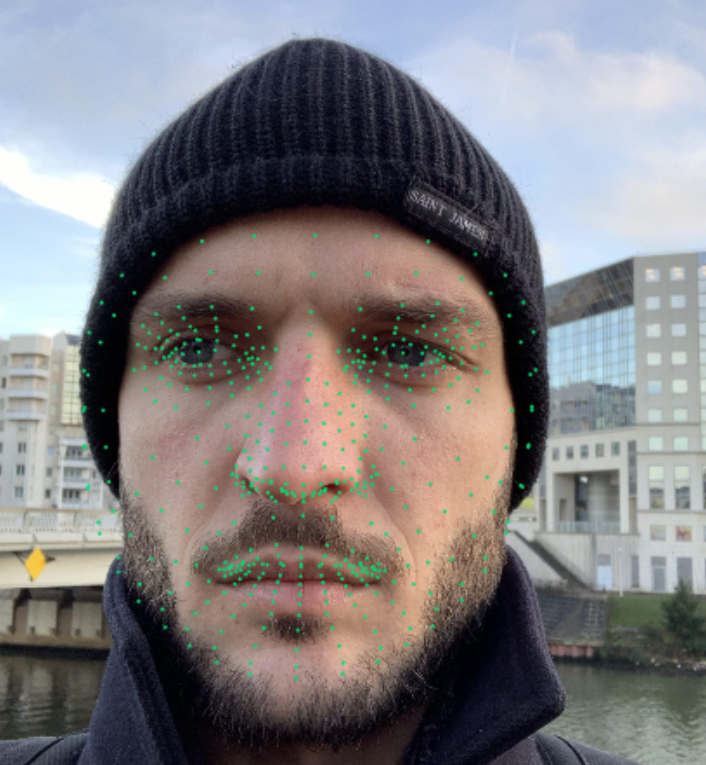# Mode Homepage# Performance

-> Model generally assumes the face will fill a significant part of the screen
-> Note this should actually create a `keypoint` type instance. However you must have a keypoint instance with a similar number of points defined in your project.

# Code

``````const model = await faceLandmarksDetection.load();
let canvas = diffgram.get_new_canvas()
const predictions = await model.estimateFaces({
input: canvas
});
console.log(predictions)
for (let i = 0; i < predictions.length; i++) {
const keypoints = predictions[i].scaledMesh;

// Log facial keypoints.
for (let i = 0; i < keypoints.length; i++) {
const [x, y, z] = keypoints[i];

//console.log(`Keypoint \${i}: [\${x}, \${y}, \${z}]`);
diffgram.create_instance_from_keypoints(x, y)
}
}
``````